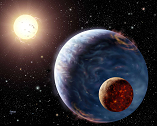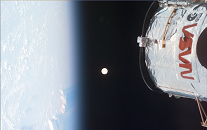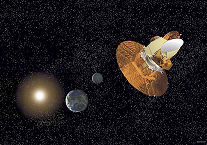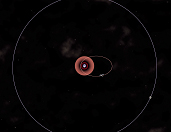Formal Education
Informal Education
Education Collaborations
Web Shortcuts
Modeling the Electromagnetic Spectrum

#### Modeling the Earth-Moon System

The Earth-Moon SystemStudents have misconceptions regarding the Earth-Moon system, since most illustrations are not accurate representations. Even though the Moon is one of the largest satellites in our Solar System, it is relatively small next to the Earth. The diameter of the Moon is one -quarter of the Earth's diameter and from this, students tend to acquire the misconception that the Moon is one-quarter the size of the Earth. The Moon is one-fiftieth the volume of the Earth; however this is not easy to visualize. In addition, the Moon's distance of thirty Earth diameters is visually impressive and usually quite a surprise to students, even if they "know the numbers" for the distance from the Earth to the Moon. The following demonstration, requiring three cans of Play-DohTM, 3.8 meters of string and a toothpick, will provide students with a powerful and accurate scale model of the Earth-Moon system.Draw a 40-cm diameter circle on the board and label it Earth. Have the students predict the size of the Moon relative to the Earth, and have some of them draw their Moons on the board. After the student predictions of Moon sizes have been discussed, explain that the true diameter of the Moon is about one-quarter of the diameter of the Earth; therefore a 40-cm diameter Earth has a 10-cm diameter Moon. Ask the students to estimate how many Moons would fit inside the Earth. Show the students 51 equal-sized balls of Play-DohTM. [Three cans of Play-DohTM with 17 balls made from each can will produce 51 balls.]Ask the students how many of the balls should be joined together to form the Earth and how many should be joined together to form the Moon. After the students have discussed possible answers, roll 50 of the balls into a single large ball. The large ball is a model of the Earth and the single remaining ball is a model of the Moon. Ask students to comment on the relative sizes of the Earth and Moon. Even though they know that the diameter of the Earth is four times the diameter of the moon, that information does not give them an understanding of the difference in volume. Explain that this is an accurate scale model of the Earth-Moon system and that fifty Moons are required to fill the same volume as the Earth. You can show the students that the moon is one-fourth the diameter of the Earth by placing the Moon across the surface of the Earth four times - demonstrating that one-fourth the diameter of the Earth is not the same as one-fourth the size of the Earth.Ask the students to estimate the distance between the Earth and Moon by indicating where the Moon should be placed relative to the Earth. After several estimates have been suggested, take a long piece of string and with one end measure the diameter of the Earth. Then fold the string back on itself repeatedly until the length is equal to thirty times the diameter of the Earth (3.81-m). Stretch out the string and then place the Moon at the end of the string. This is now an accurately scaled model of both size and distance for the Earth-Moon system. [You may decide to have a 3.8-m piece of string pre-cut and tell the students that the string is 30 Earth diameters in length.] Most students do not realize how far away the Moon is from the Earth.To further illustrate the Earth-Moon scale, ask the students how far from the Earth the space shuttles orbit. Most students will indicate that the shuttles travel a long way from Earth - as much as half the distance to the Moon. The shuttles orbit at a maximum altitude of 480-km. The Earth model that was created is approximately 12.5-cm in diameter. Insert a toothpick into the Earth model until 3.5-mm (the sharp point of the toothpick) is sticking out from the Earth. This represents the altitude at which most satellites, including the space shuttles and the Hubble Space Telescope, orbit above the Earth. The Chandra X-Ray Observatory is in a highly elliptical orbit that at closest approach is 16,000-km from Earth (1-1/4 Earth diameters or 15.6-cm) and at furthest approach is 140,000-km from Earth - approximately one-third of the distance to the Moon (1.3-m). If the students are familiar with the Apollo 13 accident, ask them what distance they estimate Apollo 13 was from the Earth when they transmitted the message: "Houston, we have a problem." Apollo 13 was approximately two thirds of the distance to the Moon (2.5-m).

Modeling the Electromagnetic Spectrum: Middle School
Modeling the Electromagnetic Spectrum: High School